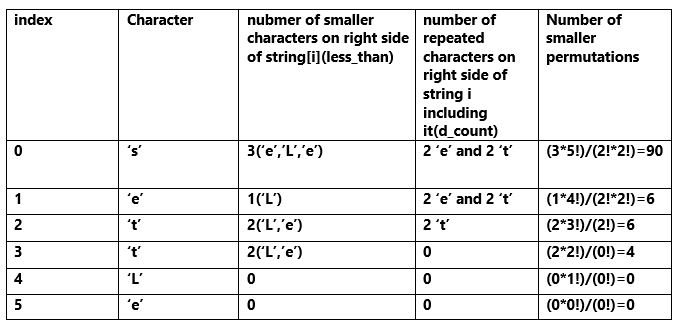# Lexicographic rank of a string with duplicate characters

Given a string s that may have duplicate characters. Find out the lexicographic rank of s. s may consist lower as well as upper case letters. We consider the lexicographic order of characters as their order of ACCII value. Hence the lexicographical order of characters will be ‘A’, ‘B’, ‘C’, …, ‘Y’, ‘Z’, ‘a’, ‘b’, ‘c’, …, ‘y’, ‘z’.

Examples:

Input : “abab”
Output : 21
Explanation: The lexicographical order is: “aabb”, “abab”, “abba”, “baab”, “baba”, “bbaa”. Hence the rank of “abab” is 2.

Input : “settLe”
Output : 107

## Recommended: Please try your approach on {IDE} first, before moving on to the solution.

Prerequisite: Lexicographic rank of a string

Method: The method here is little bit different from the without repetition version. Here we have to take care of the duplicate characters also. Let’s look at the string “settLe”. It has repetition(2 ‘e’ and 2 ‘t’) as well as upper case letter(‘L’). Total 6 characters and total number of permutations are 6!/(2!*2!).
Now there are 3 characters(2 ‘e’ and 1 ‘L’) on the right side of ‘s’ which come before ‘s’ lexicographically. If there were no repetition then there would be 3*5! smaller strings which have the first character less than ‘s’. But starting from position 0, till end there are 2 ‘s’ and 2 ‘t'(i.e. repetations). Hence number of possible smaller permutations with first letter smaller than ‘s’ are (3*5!)/(2!*2!).
Similarly if we fix ‘s’ and look at the letters from index 1 to end then there is 1 character(‘L’) lexicographically less than ‘e’. And starting from position 1 there are 2 repeated characters(2 ‘e’ and 2 ‘t’). Hence number of possible smaller permutations with first letter ‘s’ and second letter smaller than ‘e’ are (1*4!)/(2!*2!).

Similarly we can form the following table:WorkFlow:

```1. Initialize t_count(total count) variable
to 1(as rank starts from 1).
2. Run a loop for every character of the string, string[i]:
(i) using a loop count less_than(number of smaller
characters on the right side of string[i]).
(ii) take one array d_count of size 52 and using a
loop count the frequency of characters starting
from string[i].
(iii) compute the product, d_fac(the product of
factorials of each element of d_count).
(iv) compute (less_than*fac(n-i-1))/(d_fac).
3. return t_count
```

## C++

 `// C++ program to find out lexicographic ` `// rank of a string which may have duplicate ` `// characters and upper case letters. ` `#include ` `#include ` ` `  `using` `namespace` `std; ` ` `  `// Function to calculate factorial of a number. ` `int` `fac(``int` `n) ` `{ ` `    ``if` `(n == 0 or n == 1) ` `        ``return` `1; ` `    ``return` `n * fac(n - 1); ` `} ` ` `  `// Function to calculate rank of the string. ` `int` `lexRank(string s) ` `{ ` `    ``int` `n = s.size(); ` `    ``// Initialize total count to 1. ` `    ``int` `t_count = 1; ` ` `  `    ``// loop to calculate number of smaller strings. ` `    ``for` `(``int` `i = 0; i < n; i++) { ` ` `  `        ``// Count smaller characters than s[i]. ` `        ``int` `less_than = 0; ` `        ``for` `(``int` `j = i + 1; j < n; j++) { ` `            ``if` `(``int``(s[i]) > ``int``(s[j])) { ` `                ``less_than += 1; ` `            ``} ` `        ``} ` ` `  `        ``// Count frequency of duplicate characters. ` `        ``vector<``int``> d_count(52, 0); ` ` `  `        ``for` `(``int` `j = i; j < n; j++) { ` ` `  `            ``// Check whether the character is upper ` `            ``// or lower case and then increase the ` `            ``// specific element of the array. ` `            ``if` `((``int``(s[j]) >= ``'A'``) && ``int``(s[j]) <= ``'Z'``) ` `                ``d_count[``int``(s[j]) - ``'A'``] += 1; ` `            ``else` `                ``d_count[``int``(s[j]) - ``'a'` `+ 26] += 1; ` `        ``} ` ` `  `        ``// Compute the product of the factorials ` `        ``// of frequency of characters. ` `        ``int` `d_fac = 1; ` `        ``for` `(``int` `ele : d_count) ` `            ``d_fac *= fac(ele); ` ` `  `        ``// add the number of smaller string ` `        ``// possible from index i to total count. ` `        ``t_count += (fac(n - i - 1) * less_than) / d_fac; ` `    ``} ` ` `  `    ``return` `t_count; ` `} ` ` `  `// Driver Program ` `int` `main() ` `{ ` `    ``// Test case 1 ` `    ``string s1 = ``"abab"``; ` `    ``cout << ``"Rank of "` `<< s1 << ``" is: "` `         ``<< lexRank(s1) << endl; ` ` `  `    ``// Test case 2 ` `    ``string s2 = ``"settLe"``; ` `    ``cout << ``"Rank of "` `<< s2 << ``" is: "` `         ``<< lexRank(s2) << endl; ` ` `  `    ``return` `0; ` `} `

Output:

```Rank of abab is: 2
Rank of settLe is: 107
```

This algorithm runs intime.

My Personal Notes arrow_drop_upCheck out this Author's contributed articles.

If you like GeeksforGeeks and would like to contribute, you can also write an article using contribute.geeksforgeeks.org or mail your article to contribute@geeksforgeeks.org. See your article appearing on the GeeksforGeeks main page and help other Geeks.

Please Improve this article if you find anything incorrect by clicking on the "Improve Article" button below.

Article Tags :
Practice Tags :

3

Please write to us at contribute@geeksforgeeks.org to report any issue with the above content.